Courses

# Ex 6.3 NCERT Solutions- Triangles Class 10 Notes | EduRev

## Class 10 : Ex 6.3 NCERT Solutions- Triangles Class 10 Notes | EduRev

The document Ex 6.3 NCERT Solutions- Triangles Class 10 Notes | EduRev is a part of the Class 10 Course Class 10 Mathematics by VP Classes.
All you need of Class 10 at this link: Class 10

Q.1. State which pairs of triangles in Fig. are similar. Write the similarity criterion used by you for answering the question and also write the pairs of similar triangles in the symbolic form: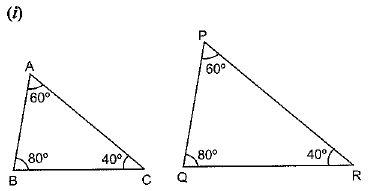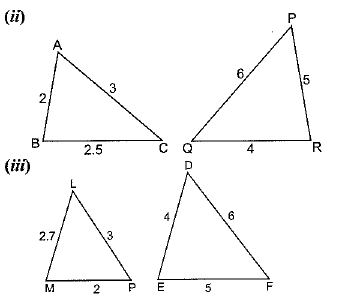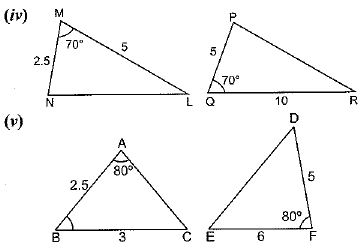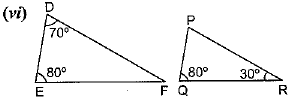Sol. (i) In Δ ABC and Δ PQR
We have:
∠A = ∠P      [Each 60°]
∠B = ∠Q      [Each 80°]
∠C = ∠R       [Each 40°]
ΔABC ~ ΔPQR     [AAA criterion]
(ii) In ΔABC and ΔQRP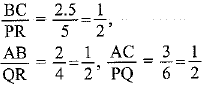Hence, ΔABC ~ ΔQRP [SSS criterion]
(iii) In ΔLMP and ΔEFD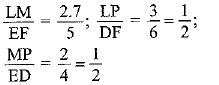∴  ΔLMP is not similar to ΔEFD.
Since the three ratios are not same.
(iv) In ΔMNL and ΔQPR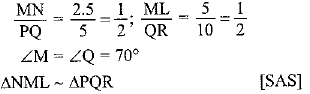(v) In ΔABC and ΔDEF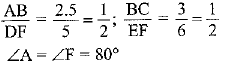ΔABC is not similar to ΔDEF
∵ Angles between two sides are not same
(vi) In ΔDEF and ΔPQR
∠E = ∠Q = 80°
∠F = ∠R = 30°
[∴ ∠F = 180° - (80° + 70°) = 30°]
∴ ΔDEF ~ ΔPQR     [AA]

Q.2. In the figure, ΔODC ~ ΔOBA, ∠BOC = 125° and ∠CDO = 70°. Find ∠DOC, ∠DCO and ∠OAB.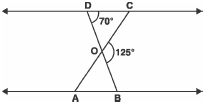Sol.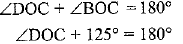⇒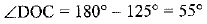In ΔDOC,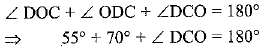⇒   125° + ∠DCO = 180°
⇒ ∠DCO = 180° - 125° = 55°
ΔODC ~ ΔOBA
∠OAB = ∠DCO = 55°
⇒ ∠DOC = ∠OAB = 55°

Q.3. Diagonals AC and BD of a trapezium ABCD with AB || DC intersect each other at the point O. Using a similarity criterion for two triangles, show that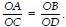Sol. Given: Diagonals AC and BD intersect at O.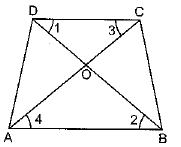AB || DC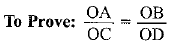Proof: In ΔAOB and ΔCOD
∠1 = ∠2
∠3 = ∠4    [Alternate angles]
∴ ΔAOB ~ ΔCOD     [AA]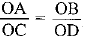[Corresponding sides of similar triangles]

Q.4. In the figure,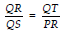and ∠1 = ∠2. Show that ΔPQS ~ ΔTQR.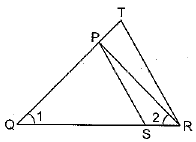Sol.  Given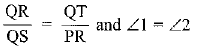To Prove: ΔPOS ∼ ΔTOR
To Prove: ΔPQS
Proof: In ΔPQR,
∠1 = ∠2    [Given]
PQ = PR  [Sides opposite to equal angles]
OR/QS = QT/PR      [Given]
Or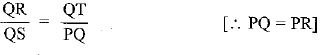In ΔPQS and ΔTQR,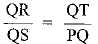(Proved)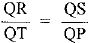∠1 = ∠1      [Common]
∴   ∠PQS ~ ∠TQR       [SAS]

Q.5. S and T are points on sides PR and QR of ΔPQR such that ∠P = ∠RTS. Show that ΔRPQ ~ ΔRTS.
Sol. In Δ RPQ ~ ΔRTS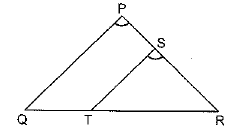∠P = ∠RTS     [Given]
∠R = ∠R       [Common]
∴ ΔRPQ ~ ΔRTS    [AA]

Q.6. In the figure, if ΔABE ≌ ΔACD, show that ΔADE ~ ΔABC.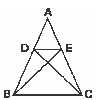Sol.
Given: ΔABE ≌ ΔACD
Proof: ΔABE ~ ΔACD
AB = AC and AE = AD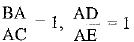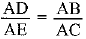[Proved above]
∠A - ∠A   [Common]

Q.7. In the figure, altitudes AD and CE of ΔABC intersect each other at the point P. Show that:
(i) ΔAEP ~ ΔCDP
(ii) ΔABD ~ ΔCBE
(iv) ΔPDC ~ ΔBEC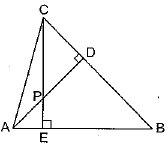Sol. Given: AD and CE are altitudes of the ΔABC
(i) To Prove: ΔAEP ~ ΔCDP
Proof: In ΔAEP and ΔCDP,
∠AEP = ∠CDP [Each'90°]
∠APE = ∠CPD [Vertically opposite angles]
ΔAEP ~ ΔCDP      [AA]
(ii) In ΔABD and ΔCBE,
∠ABD = ∠CBE    [Common]
ΔABD ~ ΔCBE    [AA]
(iii) In Δ AEP and ΔADB,
∠A = ∠A            [Common]
(iv) In ΔPDC and ΔBEC,
∠PDC = ∠BEC    [Each 90°]
∠PCD = ∠BCE    [Common]
ΔPDC ~ ΔBEC    [AA]

Q.8. E is a point on the side AD produced of a parallelogram ABCD and BE intersects CD at F. Show that Δ ABE ~ ΔCFB.
Sol.  In ΔABE and ΔCFB,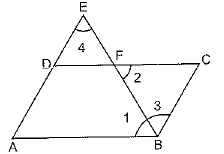∠1 = ∠2
∠4 = ∠3    [Alternate angles]
⇒ ΔABE ∼ ΔCFB     [AA]

Q.9. In the figure, ABC and AMP are two right triangles, right angled at B and M respectively. Prove that:
(i) Δ ABC ~ Δ AMP
(ii)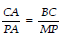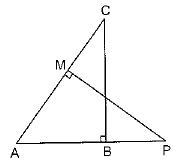Sol. (i) In ΔABC and ΔAMP,
∠B = ∠AMP [Each 90°]
∠A = ∠A [Common]
⇒ ΔABC ∼ ΔAMP [AA]
(ii) ΔABC ~ ΔAMP [proved above]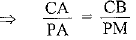[Ratio of the Corresponding sides of similar Δs]

Q.10. CD and GH are respectively the bisectors of ∠ACB and ∠EGF such that D and H lie on sides AB and FE of Δ ABC and Δ EFG respectively. If ΔABC ~ ΔFEG, show that:
(i)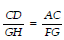(ii) ΔDCB ~ ΔHGE
(iii) ΔDCA ~ ΔHGF
Sol.  ΔABC ~ ΔFEG     [Given]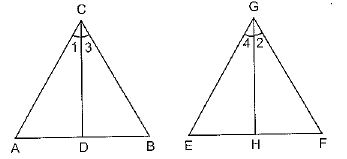⇒ ∠A = ∠F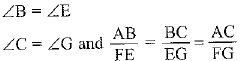(i) In ΔACD and ΔFGH
∠A = ∠F    [Given]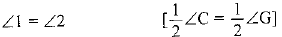∴  ΔACD ~ ΔFGH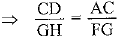[Corresponding sides of similar triangles]
(ii)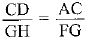[Proved above]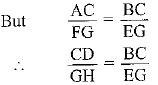In ΔDCB and ΔHGE,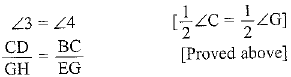∴  ΔDCB ~ ΔHGE       [SAS]

(iii) In ΔDCA and ΔHGF,
∠1 = ∠2          [Bisectors]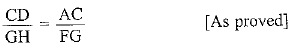⇒ ΔDCA ~ ΔHGF    [SAS]

Q.11. In the figure, E is a point on side CB produced of an isosceles triangle ABC with AB = AC. If AD ⊥BC and EF ⊥AC, prove that ΔABD ~ ΔECF.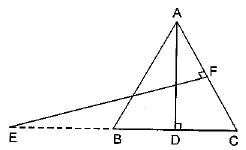Sol.  In ΔABD and ΔECF,
∠B = ∠C
[angles opposite to equal sides are equal]
⇒ ΔABD ~ ΔECF     [AA]

Q.12. Sides AB and BC and median AD of a triangle ABC are respectively proportional to sides PQ and QR and median PM of Δ PQR (see figure). Show that Δ ABC ~ ΔPQR.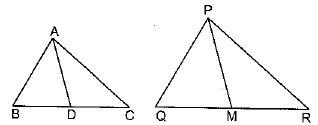Sol. In ΔABC and ΔPQR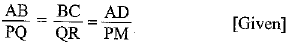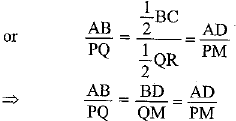⇒ ΔABD ~ ΔPQM     [SAS]
∴ ∠B = ∠Q
[Corresponding angles of similar triangles]
In ΔABC and ΔPQR,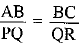[Given]
∠B = ∠Q    [As provecd]
∴  ΔABC ~ ΔPQR    [SAS]

Q.13. D is a point on the side BC of a triangle ABC such that ∠ADC = ∠BAC. Show that CA2 = CB.CD.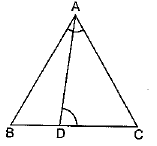Sol. In ΔACB and ΔDCA,
∠C = ∠C       [Common]
ΔACB ~ ΔDCA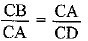[Corresponding sides of similar triangles]
⇒ CA2 = CB x CD

Q.14. Sides AB and AC and median AD of a triangle ABC are respectively proportional to sides PQ and PR and median PM of another triangle PQR. Show that ΔABC ~ ΔPQR.
Sol. Construction: Draw DE || AC and MS || PR
Proof: In ΔABC, D is mid-point of BC (given) and DE || AC (const.)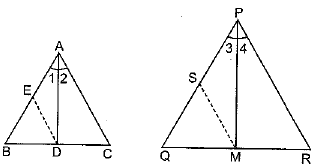∴ E is mid-point of AB (by converse of mid-point theorem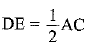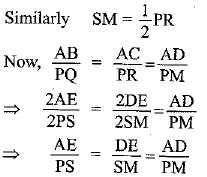∴ ΔADE ~ ΔPMS [SSS similarly]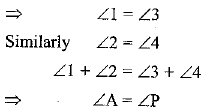Now, in ΔABC and ΔPQR,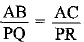[Given]
∠A = ∠P    [Proved above]
∴ ΔABC ~ ΔPQR   (SAS)

Q.15. A vertical pole of length 6 m casts a shadow 4 m long on the ground and at the same time a tower casts a shadow 28 m long. Find the height of the tower.
Sol. DE is vertical stick of length = 6m
Length of shadow = 4 m
Let height of tower = h m
Length of shadow = 28 m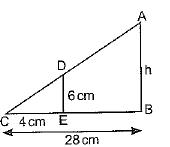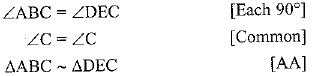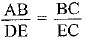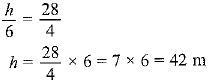Q.16. If AD and PM are medians of triangles ABC and PQR, respectively where ΔABC ~ ΔPQR, prove that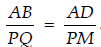.
Sol. When ΔABC ~ ΔPQR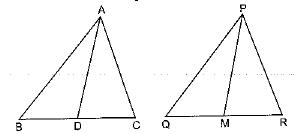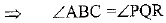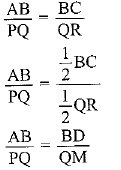In ΔABD and ΔPQM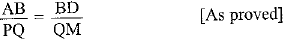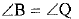∴  ΔABD ~ ΔPQM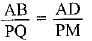[Corresponding sides of similar triangles]

Offer running on EduRev: Apply code STAYHOME200 to get INR 200 off on our premium plan EduRev Infinity!

132 docs

,

,

,

,

,

,

,

,

,

,

,

,

,

,

,

,

,

,

,

,

,

;